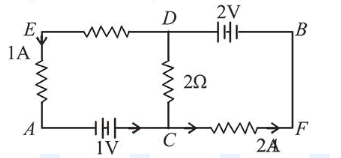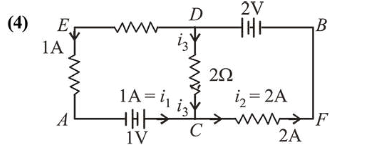# In the circuit, given in the figure currents in different branches and value of one resistor are shown.Question:

In the circuit, given in the figure currents in different branches and value of one resistor are shown. Then potential at point $B$ with respect to the point $A$ is :1. (1) $+2 \mathrm{~V}$

2. (2) $-2 \mathrm{~V}$

3. (3) $-1 \mathrm{~V}$

4. (4) $+1 V$

Correct Option: , 4

Solution:Let us assume the potential at $A=V_{A}=0$

Using Kirchoff's junction rule at $C$, we get

$i_{1}+i_{3}=i_{2}$

$1 \mathrm{~A}+i_{3}=2 \mathrm{~A} \Rightarrow i_{3}=2 \mathrm{~A}$

Now using Kirchoff's loop law along $A C D B$

$V_{A}+1+i_{3}(2)-2=V_{B}$

$\Rightarrow V_{A}+1+i_{3}(1)-2=V_{B}$

$\Rightarrow V_{B}-V_{A}=3-2=1$ volt